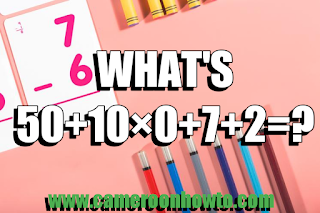050+10×0 +7+2=? Answer

Welcome to Cameroonhowto, your Education, business, Scholarship and tech site. Today I will solve this trending Math Quiz that's making many people scratch their heads.

This particular Quiz is tricky, if you are too quick, you might fail it. Because you learn in primary school that anything Multiply by Zero is zero, that's why you will get this quiz wrong in a glance.

The aboves statement works only when there's a bracket and the variable is Multiplying everything in the Bracket.

## What's 50 + 10 × 0 + 7 + 2 = ? Answer

The correct answer would be 59 not 9. The Quiz is probably to test your primary school Math. I will solve it using BODMAS.

BODMAS is acronym which stands for Bracket

Order of Operations

Division

Multiplication

Subtraction

Applying BODMAS, there's no Bracket.

So, the first thing to solve is 10 × 0 = 0.

Now arranging the question,

50 + 0 + 7 + 2=?

59 =?

Therefore the correct answer to"Please don't use a calculator" What's 50 + 10 × 0 + 7 + 2 = ? is math quiz 59

Also check: 18÷ 3(5-2) = ? Answer

Do you have another answer or another way to get this answer? Share with us

Tags